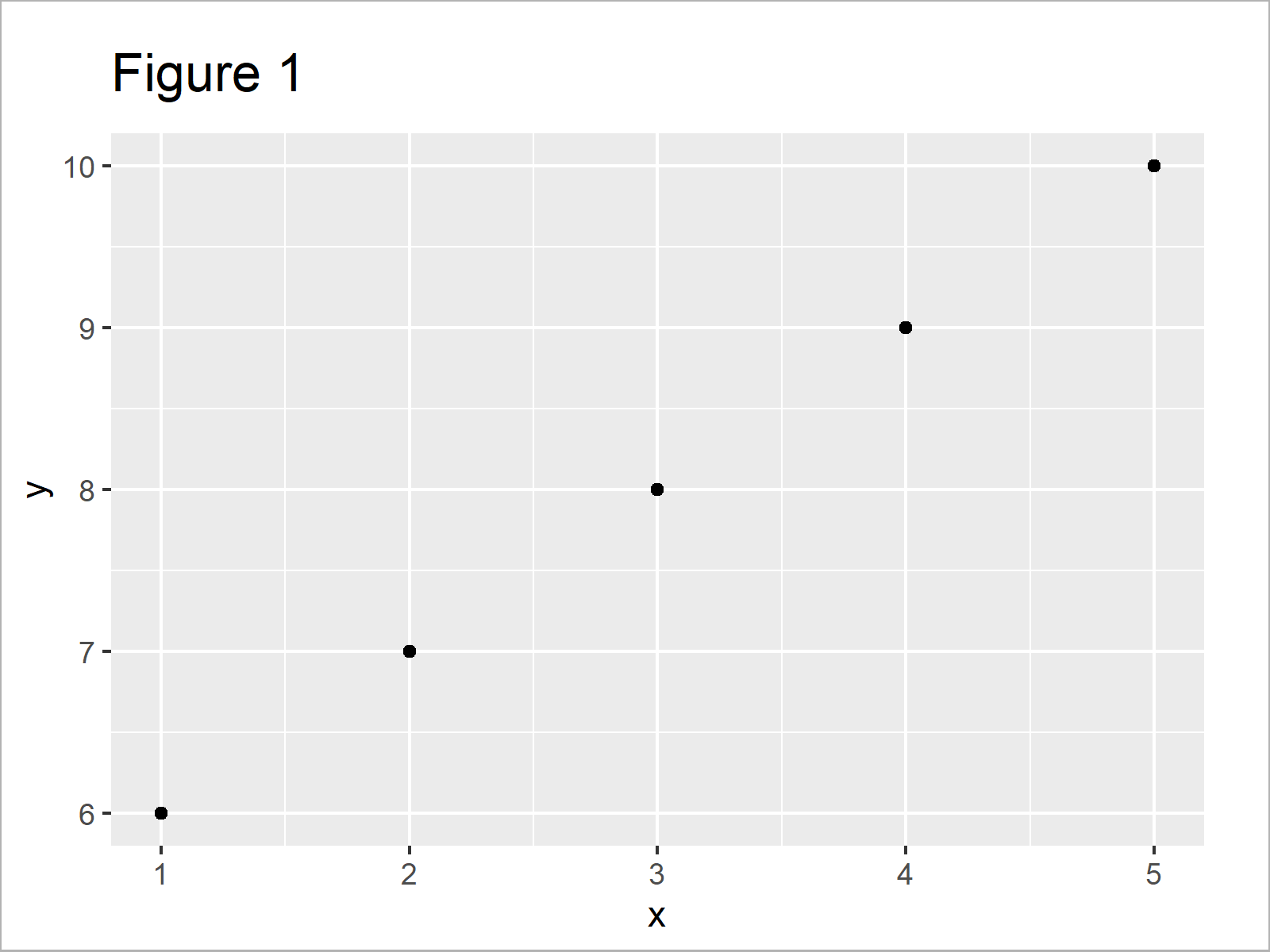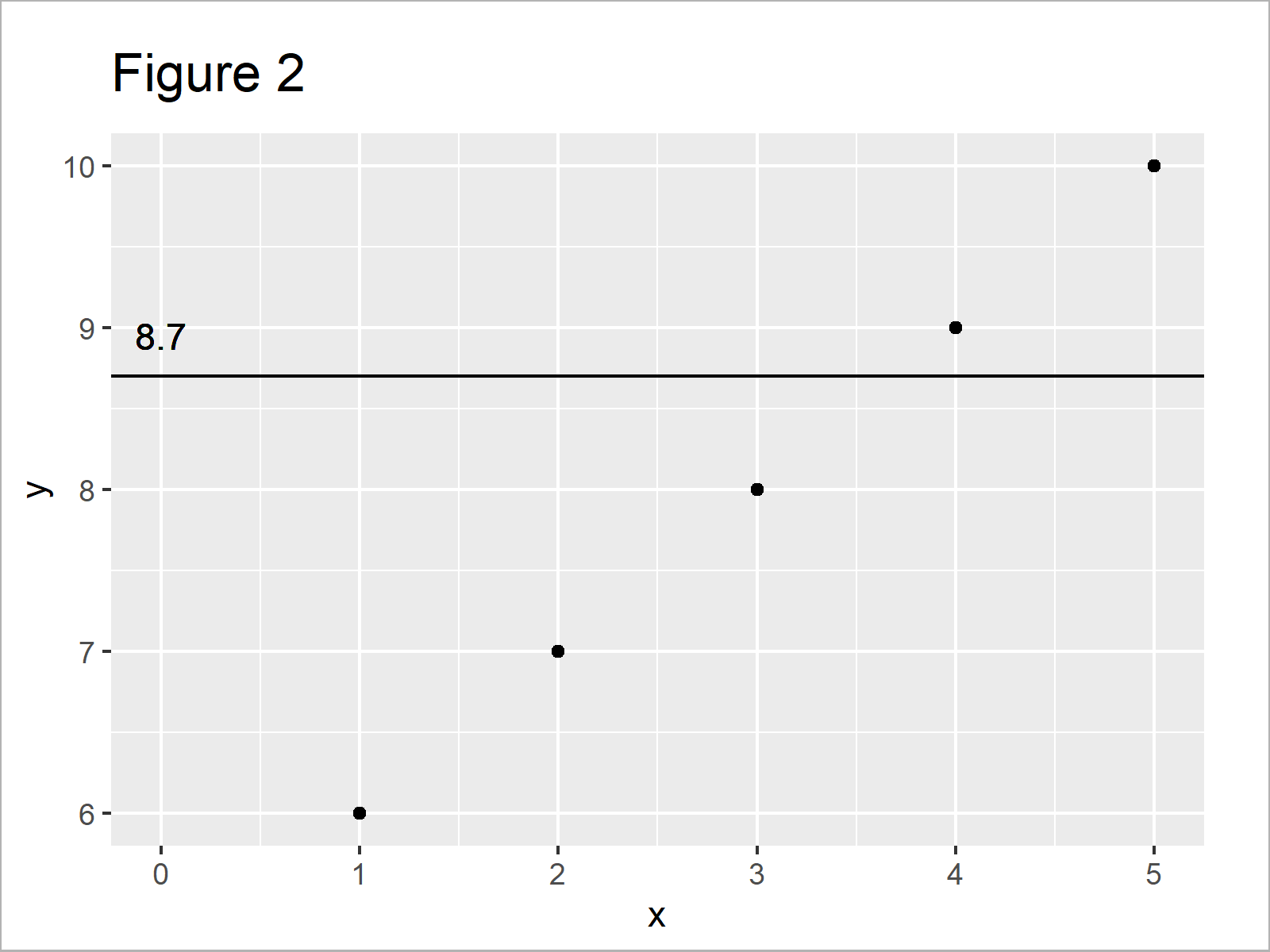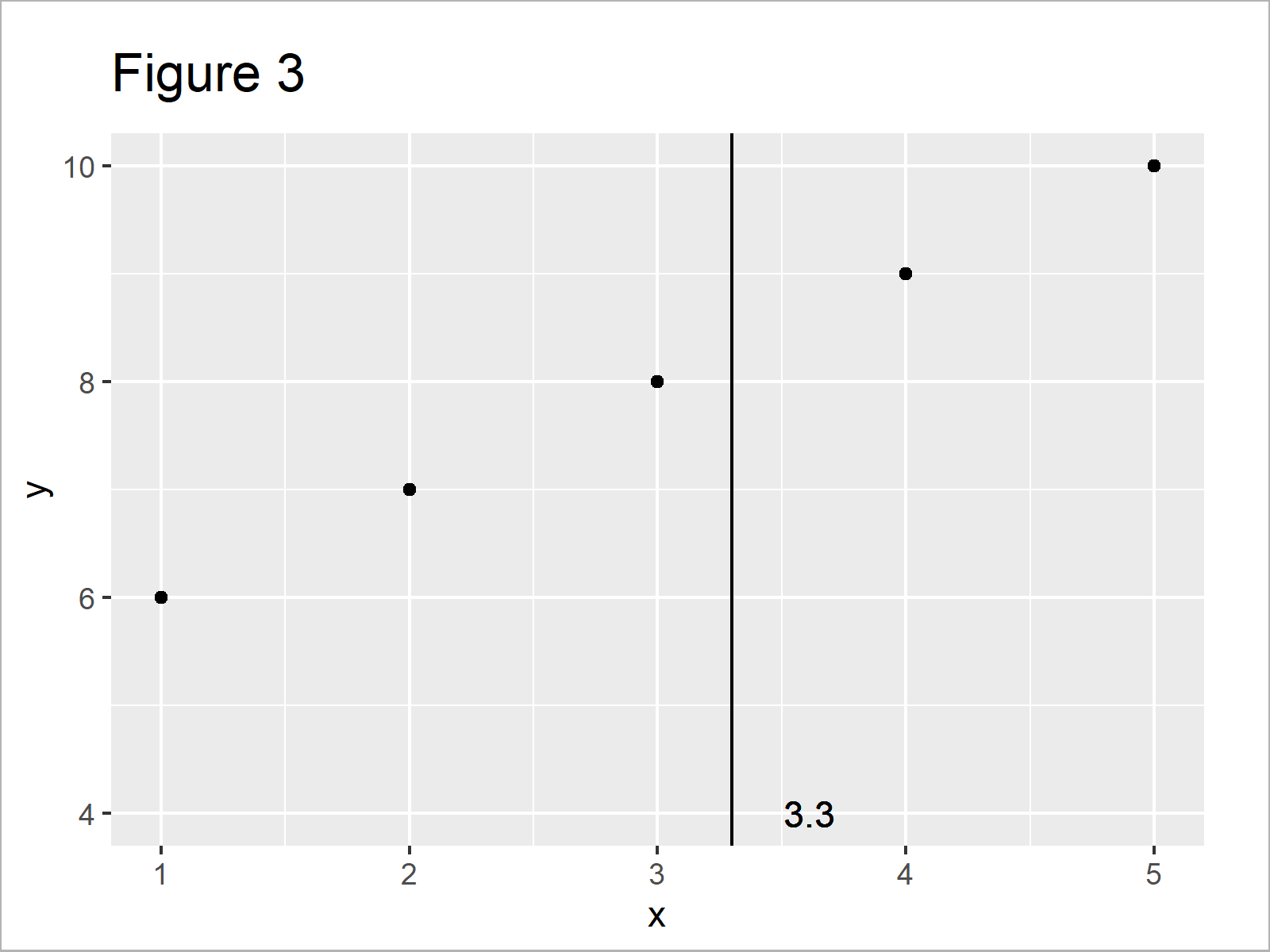# Add Label to Straight Line in ggplot2 Plot in R (2 Examples)

In this article you’ll learn how to add a labeled line to a ggplot2 graphic in R programming.

The post contains the following contents:

Let’s dive into it.

## Example Data, Add-On Packages & Default Graphic

We use the following data as basement for this R programming tutorial:

```data <- data.frame(x = 1:5,         # Create example data
y = 6:10)
data                                # Print example data```Table 1 illustrates the structure of our example data – It consists of five lines and two integer columns.

In order to use the functions of the ggplot2 package, we also have to install and load ggplot2:

```install.packages("ggplot2")         # Install ggplot2 package

Now, we can plot our data as follows:

```ggp <- ggplot(data, aes(x, y)) +    # Create plot without line
geom_point()
ggp                                 # Draw plot without line```As shown in Figure 1, the previously shown syntax has created a ggplot2 scatterplot without any lines or labels.

## Example 1: Labeling a Horizontal Line in a ggplot2 Plot

This example explains how to add a straight horizontal line with a label to our ggplot2 plot.

First, we have to define the location on the y-axis of our straight line:

`h_line <- 8.7                       # Position of horizontal line`

Next, we can use the geom_hline and geom_text functions to add a straight line with a text label to our ggplot2 graphic:

```ggp +                               # Add horizontal line & label
geom_hline(aes(yintercept = h_line)) +
geom_text(aes(0, h_line, label = h_line, vjust = - 1))```By running the previous R programming syntax we have created Figure 2, i.e. a ggplot2 scatterplot with straight line and label.

## Example 2: Labeling a Vertical Line in a ggplot2 Plot

This section demonstrates how to add a straight vertical line with label to a ggplot2 graph.

As in the previous example, we first have to set the position of our line:

`v_line <- 3.3                       # Position of vertical line`

Now, we can apply the geom_vline and geom_text functions to add our vertical line with label:

```ggp +                               # Add vertical line & label
geom_vline(aes(xintercept = v_line)) +
geom_text(aes(v_line, 4, label = v_line, hjust = - 1))```## Video & Further Resources

Do you want to know more about lines in ggplot2 graphs? Then you might want to watch the following video of my YouTube channel. In the video, I’m explaining the contents of this post in R.

Please accept YouTube cookies to play this video. By accepting you will be accessing content from YouTube, a service provided by an external third party.If you accept this notice, your choice will be saved and the page will refresh.

Furthermore, you could have a look at some of the related articles on this website:

In summary: In this R programming tutorial you have learned how to draw a straight labeled line in a ggplot2 plot. Please let me know in the comments section, in case you have any further questions.

Subscribe to the Statistics Globe Newsletter